Скачать презентацию Work Power Potential Energy Kinetic Energy 100 100

397ef2b50974d54cedd8f05740f94979.ppt

• Количество слайдов: 22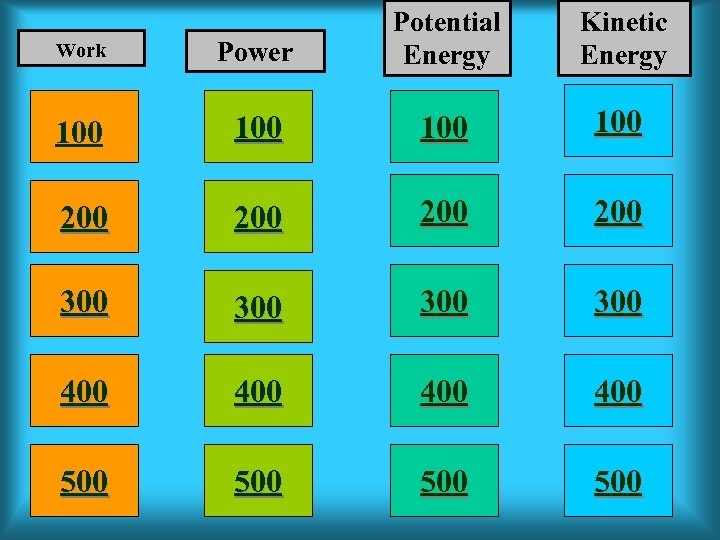Work Power Potential Energy Kinetic Energy 100 100 200 200 300 300 400 400 500 500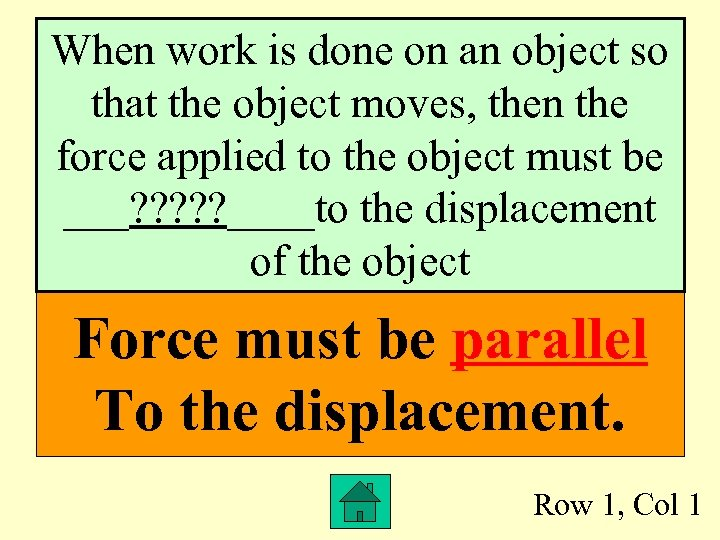When work is done on an object so that the object moves, then the force applied to the object must be ___? ? ? ____to the displacement of the object Force must be parallel To the displacement. Row 1, Col 1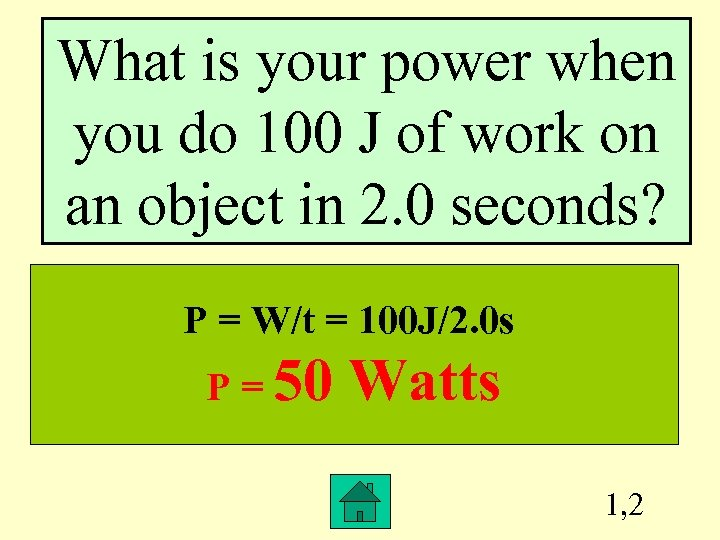What is your power when you do 100 J of work on an object in 2. 0 seconds? P = W/t = 100 J/2. 0 s P = 50 Watts 1, 2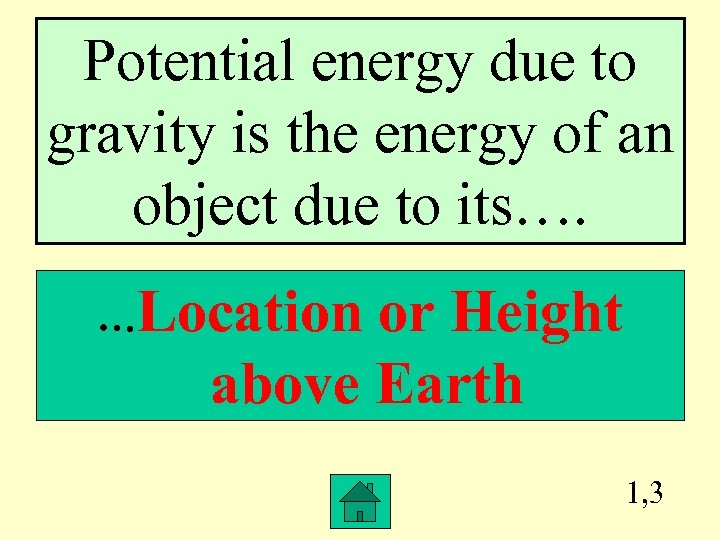Potential energy due to gravity is the energy of an object due to its…. …Location or Height above Earth 1, 3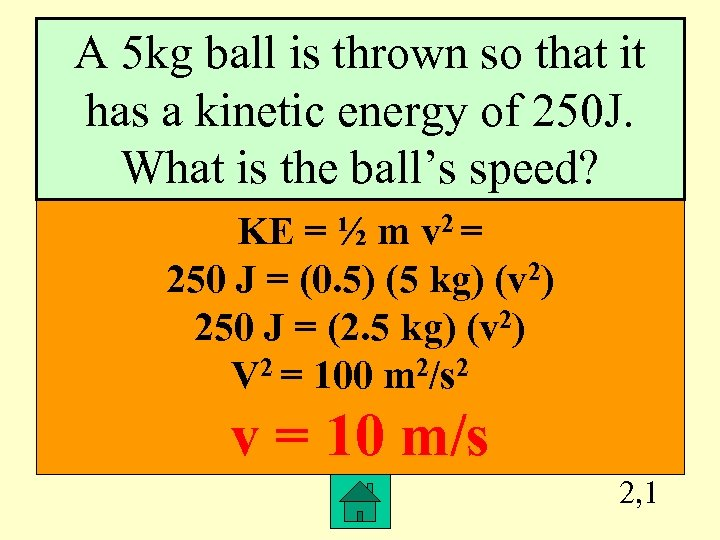A 5 kg ball is thrown so that it has a kinetic energy of 250 J. What is the ball’s speed? KE = ½ m v 2 = 250 J = (0. 5) (5 kg) (v 2) 250 J = (2. 5 kg) (v 2) V 2 = 100 m 2/s 2 v = 10 m/s 2, 1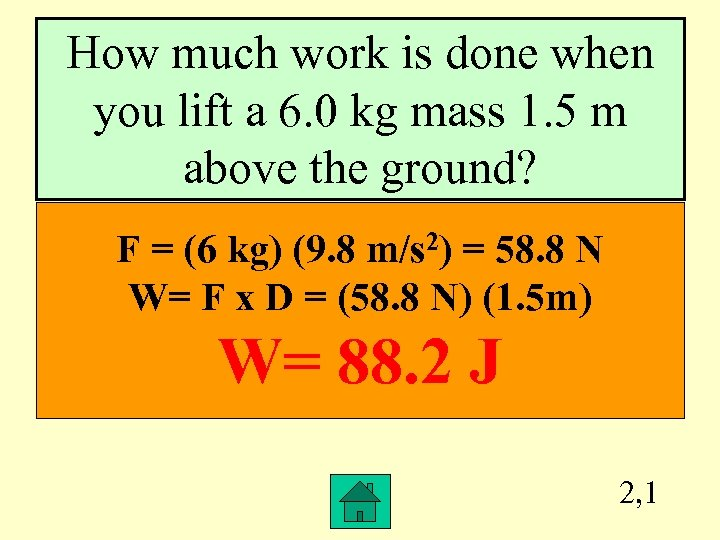How much work is done when you lift a 6. 0 kg mass 1. 5 m above the ground? F = (6 kg) (9. 8 m/s 2) = 58. 8 N W= F x D = (58. 8 N) (1. 5 m) W= 88. 2 J 2, 1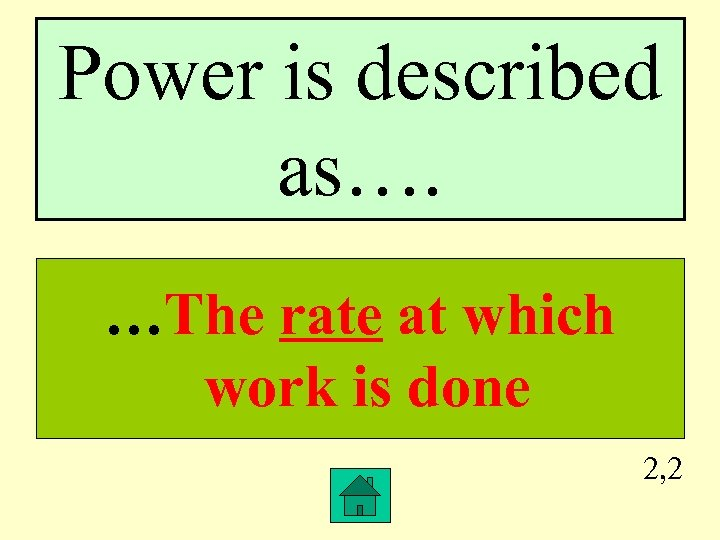Power is described as…. …The rate at which work is done 2, 2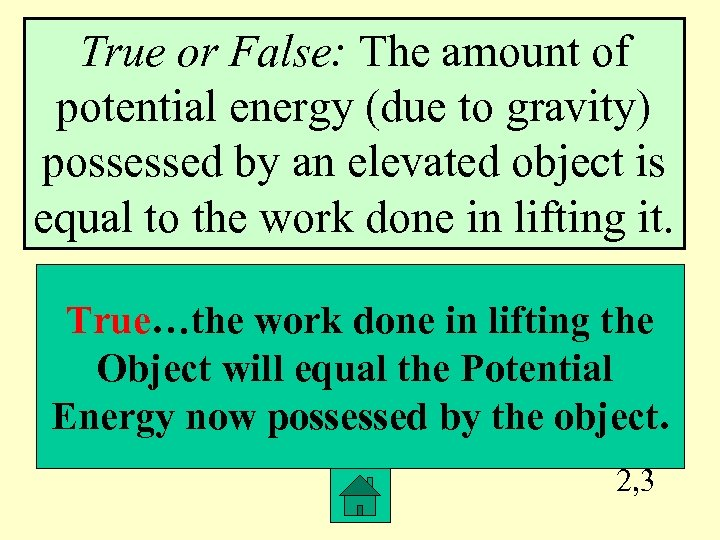True or False: The amount of potential energy (due to gravity) possessed by an elevated object is equal to the work done in lifting it. True…the work done in lifting the Object will equal the Potential Energy now possessed by the object. 2, 3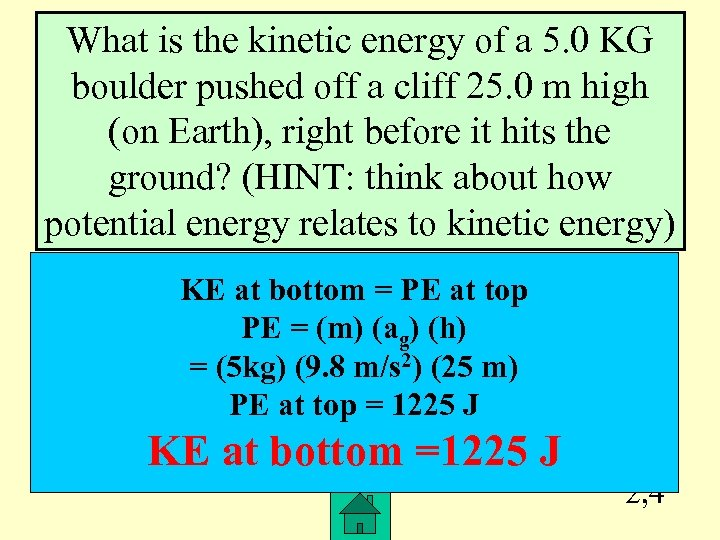What is the kinetic energy of a 5. 0 KG boulder pushed off a cliff 25. 0 m high (on Earth), right before it hits the ground? (HINT: think about how potential energy relates to kinetic energy) KE at bottom = PE at top PE = (m) (ag) (h) = (5 kg) (9. 8 m/s 2) (25 m) PE at top = 1225 J KE at bottom =1225 J 2, 4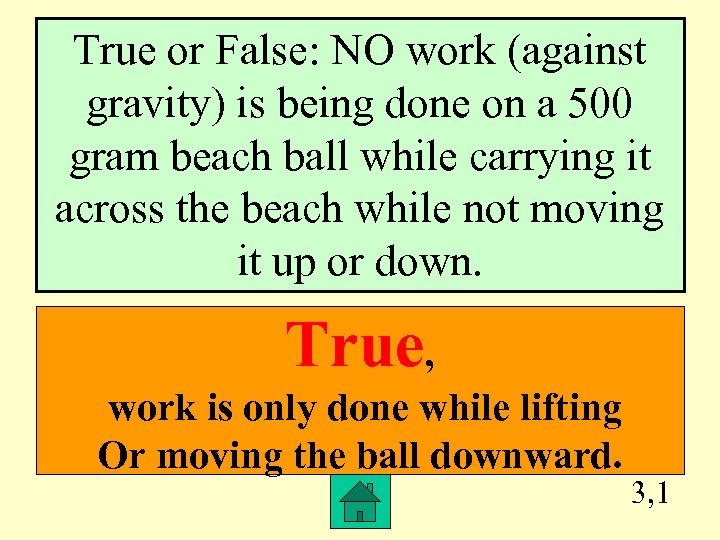True or False: NO work (against gravity) is being done on a 500 gram beach ball while carrying it across the beach while not moving it up or down. True, work is only done while lifting Or moving the ball downward. 3, 1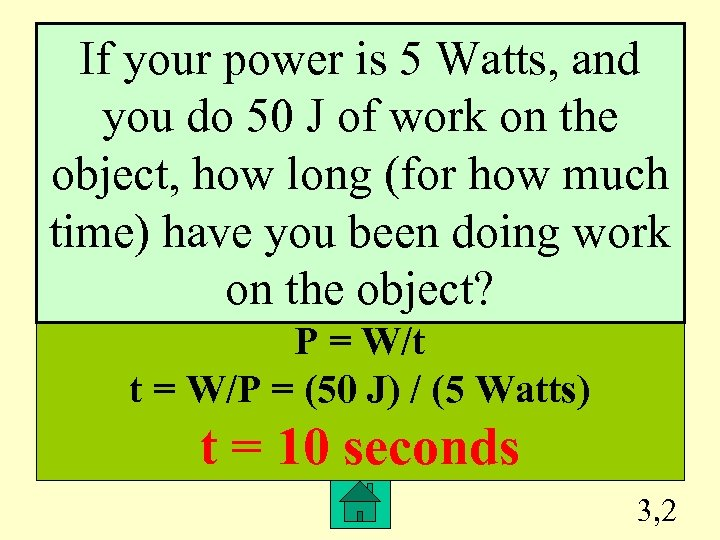If your power is 5 Watts, and you do 50 J of work on the object, how long (for how much time) have you been doing work on the object? P = W/t t = W/P = (50 J) / (5 Watts) t = 10 seconds 3, 2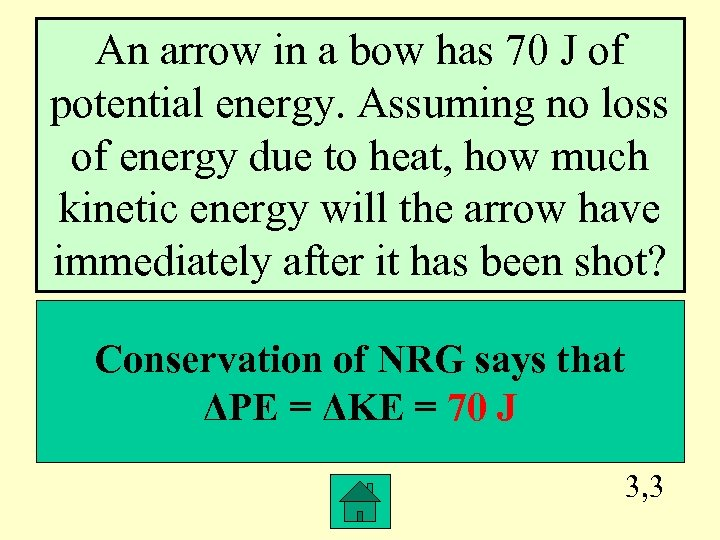An arrow in a bow has 70 J of potential energy. Assuming no loss of energy due to heat, how much kinetic energy will the arrow have immediately after it has been shot? Conservation of NRG says that ΔPE = ΔKE = 70 J 3, 3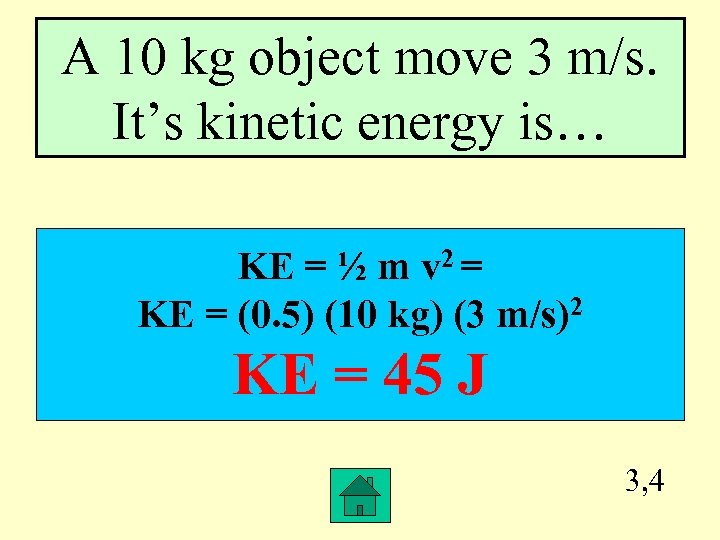A 10 kg object move 3 m/s. It’s kinetic energy is… KE = ½ m v 2 = KE = (0. 5) (10 kg) (3 m/s)2 KE = 45 J 3, 4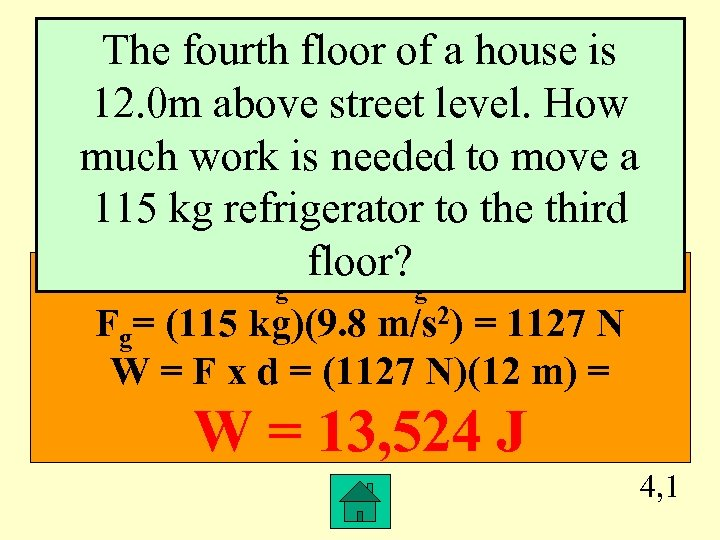The fourth floor of a house is 12. 0 m above street level. How much work is needed to move a 115 kg refrigerator to the third F =floor? = mxa g g Fg= (115 kg)(9. 8 m/s 2) = 1127 N W = F x d = (1127 N)(12 m) = W = 13, 524 J 4, 1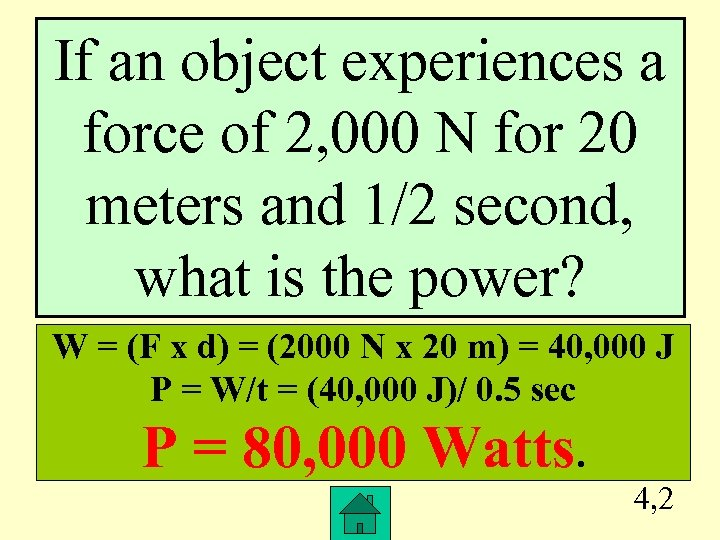If an object experiences a force of 2, 000 N for 20 meters and 1/2 second, what is the power? W = (F x d) = (2000 N x 20 m) = 40, 000 J P = W/t = (40, 000 J)/ 0. 5 sec P = 80, 000 Watts. 4, 2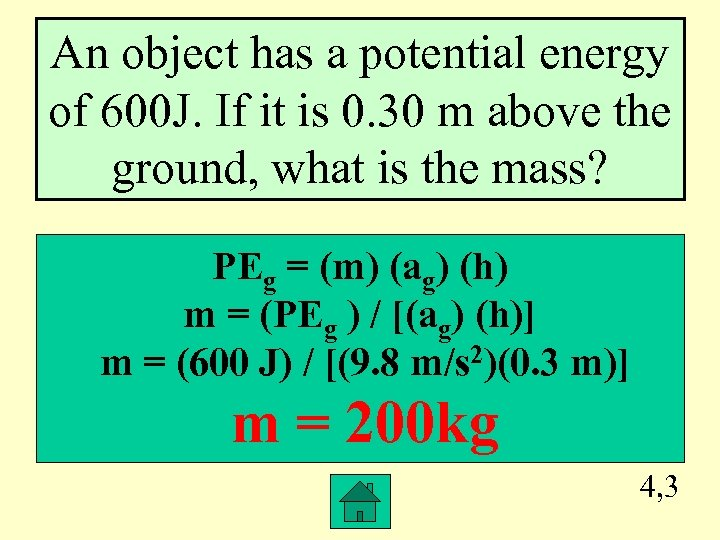An object has a potential energy of 600 J. If it is 0. 30 m above the ground, what is the mass? PEg = (m) (ag) (h) m = (PEg ) / [(ag) (h)] m = (600 J) / [(9. 8 m/s 2)(0. 3 m)] m = 200 kg 4, 3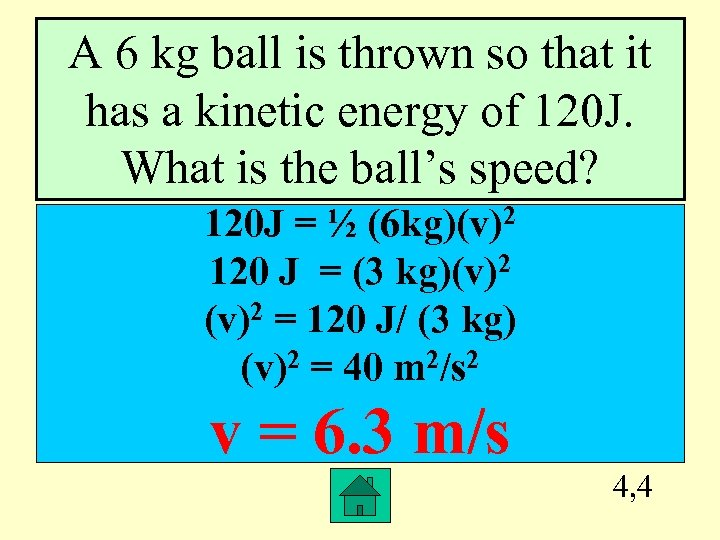A 6 kg ball is thrown so that it has a kinetic energy of 120 J. What is the ball’s speed? 120 J = ½ (6 kg)(v)2 120 J = (3 kg)(v)2 = 120 J/ (3 kg) (v)2 = 40 m 2/s 2 v = 6. 3 m/s 4, 4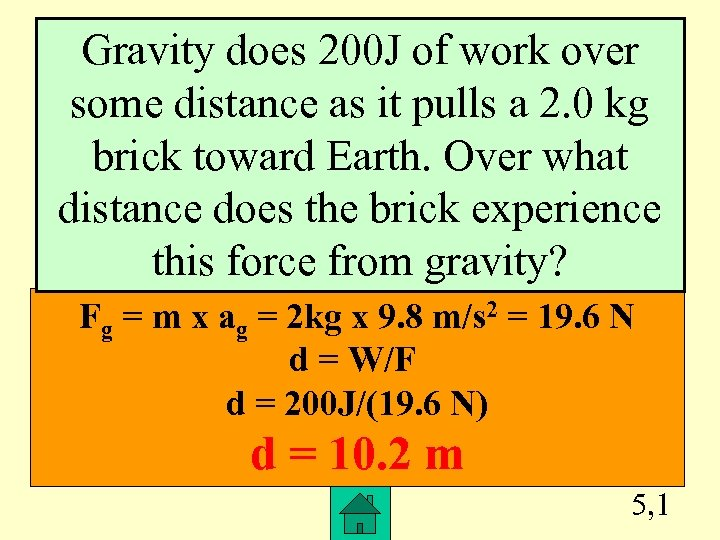Gravity does 200 J of work over some distance as it pulls a 2. 0 kg brick toward Earth. Over what distance does the brick experience this force from gravity? Fg = m x ag = 2 kg x 9. 8 m/s 2 = 19. 6 N d = W/F d = 200 J/(19. 6 N) d = 10. 2 m 5, 1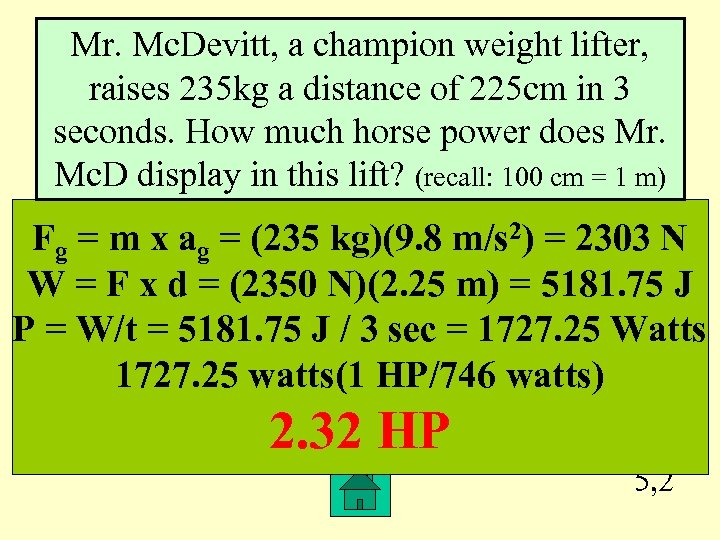Mr. Mc. Devitt, a champion weight lifter, raises 235 kg a distance of 225 cm in 3 seconds. How much horse power does Mr. Mc. D display in this lift? (recall: 100 cm = 1 m) Fg = m x ag = (235 kg)(9. 8 m/s 2) = 2303 N W = F x d = (2350 N)(2. 25 m) = 5181. 75 J P = W/t = 5181. 75 J / 3 sec = 1727. 25 Watts 1727. 25 watts(1 HP/746 watts) 2. 32 HP 5, 2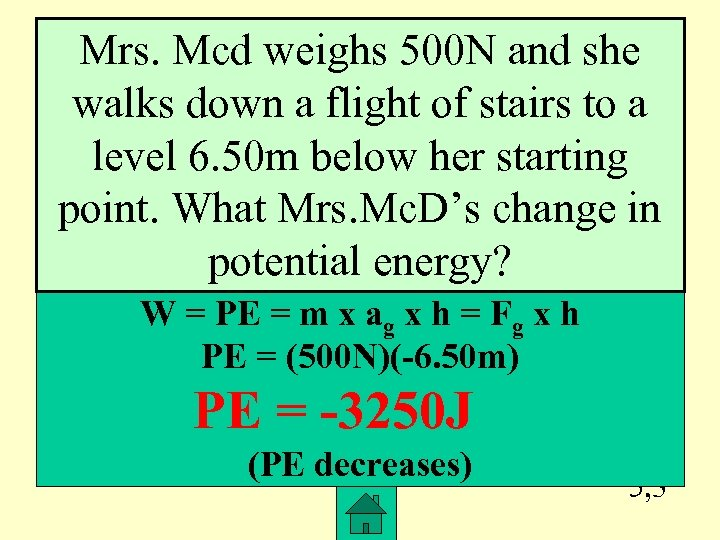Mrs. Mcd weighs 500 N and she walks down a flight of stairs to a level 6. 50 m below her starting point. What Mrs. Mc. D’s change in potential energy? W = PE = m x ag x h = Fg x h PE = (500 N)(-6. 50 m) PE = -3250 J (PE decreases) 5, 3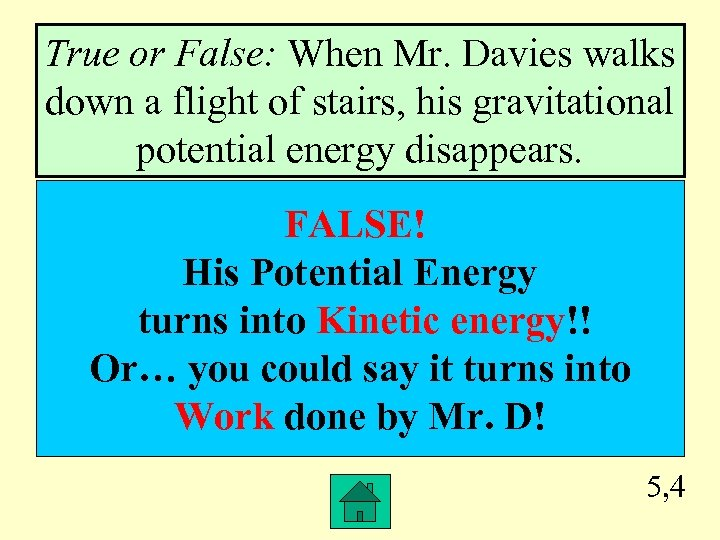True or False: When Mr. Davies walks down a flight of stairs, his gravitational potential energy disappears. FALSE! His Potential Energy turns into Kinetic energy!! Or… you could say it turns into Work done by Mr. D! 5, 4